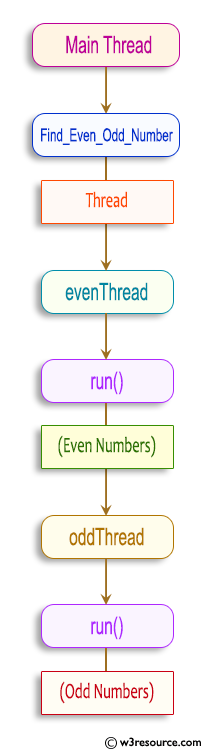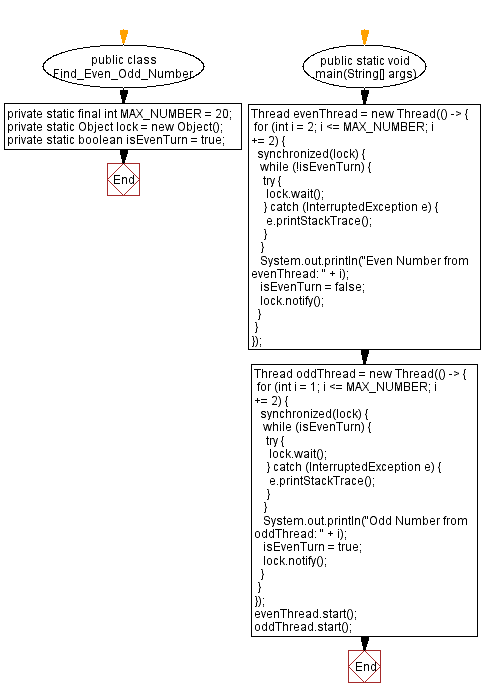﻿ Java Program: Find and Print Even-Odd Numbers with Threads

# Java thread Programming - Find and Print Even-Odd Numbers with Threads

## Java Thread: Exercise-2 with Solution

Write a Java program that creates two threads to find and print even and odd numbers from 1 to 20.

Sample Solution:

Java Code:

``````public class Find_Even_Odd_Number {
private static final int MAX_NUMBER = 20;
private static Object lock = new Object();
private static boolean isEvenTurn = true;

public static void main(String[] args) {
for (int i = 2; i <= MAX_NUMBER; i += 2) {
synchronized(lock) {
while (!isEvenTurn) {
try {
lock.wait();
} catch (InterruptedException e) {
e.printStackTrace();
}
}
System.out.println("Even Number from evenThread: " + i);
isEvenTurn = false;
lock.notify();
}
}
});

for (int i = 1; i <= MAX_NUMBER; i += 2) {
synchronized(lock) {
while (isEvenTurn) {
try {
lock.wait();
} catch (InterruptedException e) {
e.printStackTrace();
}
}
System.out.println("Odd Number from oddThread: " + i);
isEvenTurn = true;
lock.notify();
}
}
});

}
}
```
```

Sample Output:

``` Even Number from evenThread: 2
```Explanation:

The evenThread prints even numbers. It starts with 2 and increments by 2 in each iteration until it reaches MAX_NUMBER (which is 20 in this case). It uses a synchronized block with a while loop to check if it's its turn to print. If not, it waits by calling lock.wait(). When it's its turn, it prints the even number and notifies the oddThread by calling lock.notify().

The oddThread prints odd numbers. It starts with 1 and increments by 2 in each iteration until it reaches MAX_NUMBER. Similar to the evenThread, it uses a synchronized block with a while loop to check if it's its turn to print. If not, it waits by calling lock.wait(). When it's its turn, it prints the odd number and notifies the evenThread by calling lock.notify().

Flowchart:Java Code Editor:

Improve this sample solution and post your code through Disqus

What is the difficulty level of this exercise?

Test your Programming skills with w3resource's quiz.

﻿

## Java: Tips of the Day

Converts a string from camelcase:

```public static String fromCamelCase(String input, String separator) {
return input
.replaceAll("([a-z\\d])([A-Z])", "\$1" + separator + "\$2")
.toLowerCase();
}
```

Ref: https://bit.ly/3u09A9E

We are closing our Disqus commenting system for some maintenanace issues. You may write to us at reach[at]yahoo[dot]com or visit us at Facebook